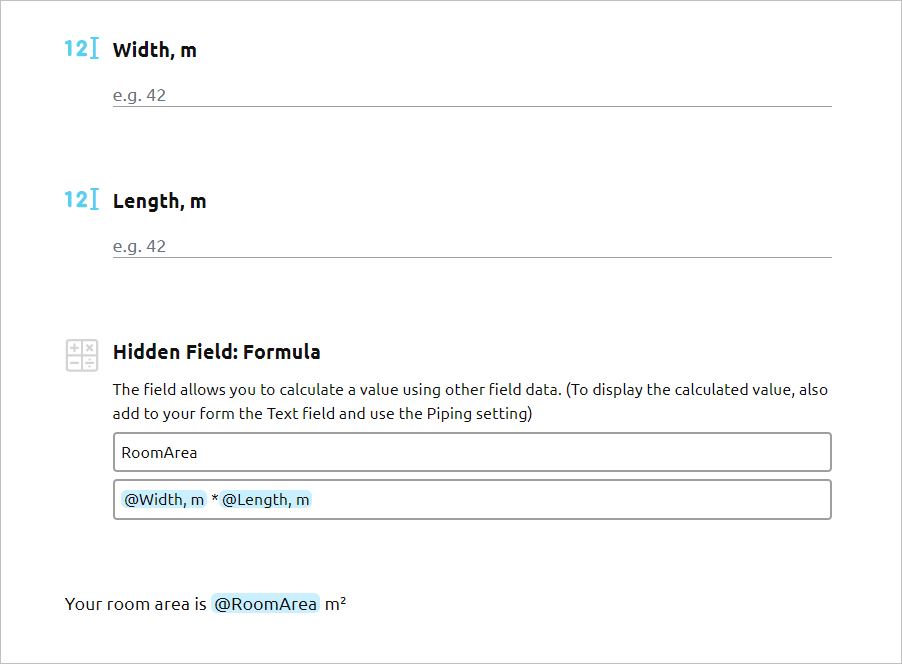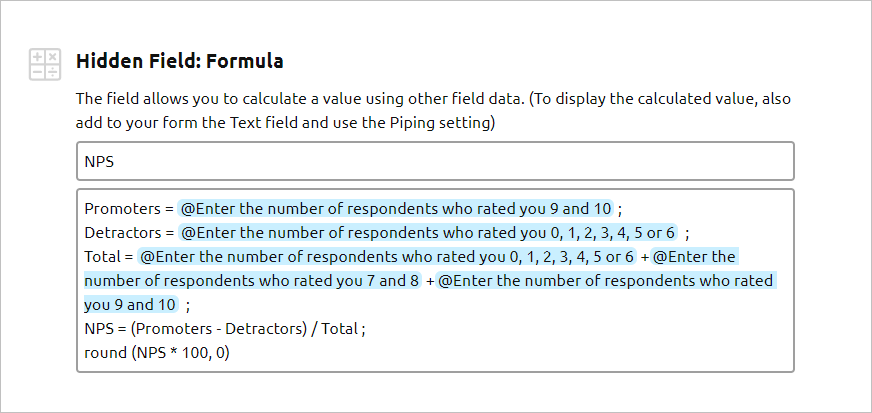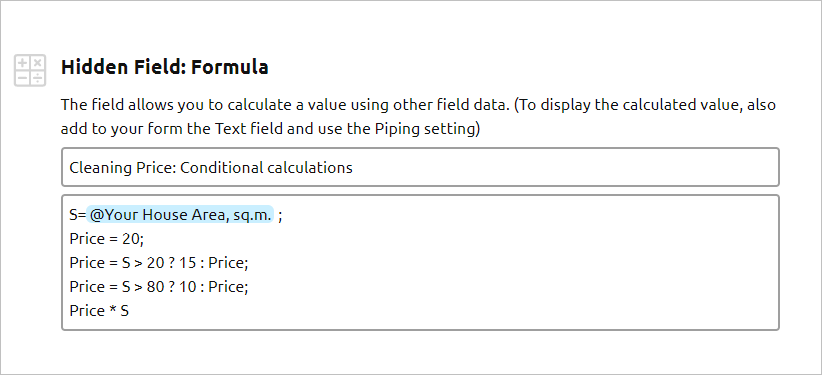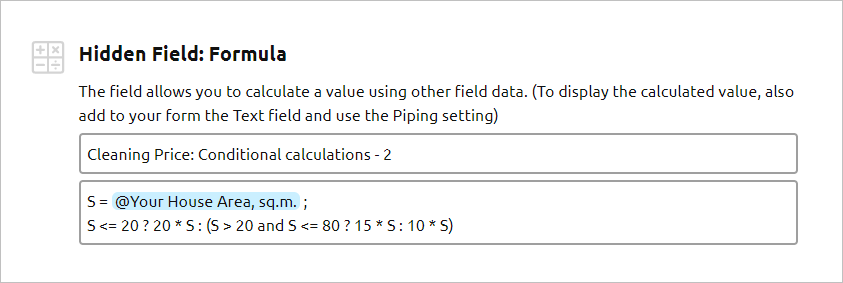# How to Use the Formula field (Expert and Ultimate tariffs)

The Formula field is a hidden field that is not displayed on the published form. The field allows you to set up formulas and calculate a value using figures that your respondents enter into different fields on your form. To display the result of the calculation, you need to use the piping functionality (@) in the Text form fields. One form can have multiple Formula fields.

## What is the difference between the Formula field and the Calculation field?

The Formula field only works with numbers! The field allows you to set a mathematical expression that will use numbers that your respondents enter in other fields on your form.

The Calculation field allows you to calculate a value based on the conditions that you set for other fields. For example, you have a multiple-choice field with Option 1 and Option 2. If your respondent chooses Option 1, the Calculation adds 1 to the total, if your respondent chooses Option 2, the Calculation adds 10 to the total. Read more about the Calculation field

## The Formula field allows you to:

1. Perform mathematical calculations according to the set formula.
2. Format the calculation result, using the functions from the Math.js library, for example, rounding numbers, etc.
3. Create multi-statement calculations.
4. Make conditional calculations.

## How to set up the Formula field and display the calculation result.

1. Add the Formula form element to any part of your created form.
2. Enter the field name.
3. Enter your desired formula. To use the data from your form fields, use the @ function to reference the fields in the formula.
4. Add the Text field to your form. Type in the text you would like to display for your respondents and use the @ function to reference the Formula field result.

Example: your form calculates a room area using the data it collects from two form fields: Width, m and Length, m

The screenshot below illustrates the Area Calculator setup.## How to round your Formula field result:

The round function allows you to round your formula result with specified precision — zero, one, two, etc. decimals displayed.

For example, you are going to add numbers from two form fields and round the result of the calculation:

Example 1: round(@field1 + @field2, 3) This will return the result with three decimals.

Example 2: round(@field1 + @field2, 0) This will return the result with zero decimals.

The ´round´ function is just one of the functions from the Math.js library that is supported by AidaForm. To review the full list, please visit https://mathjs.org/docs/reference/functions.html

## How to make multi-statement calculations

Sometimes you may want to split your formula into several lines (separate statements or calculations) to make it easier to work with.

Important: Use semicolons to separate statements!!!

Example: to calculate the Net Promoter Score, we decided 1) to replace the long field labels employed in the formula with assignments and 2) to split the formula calculations into several parts.

As a result, the calculations become easier to read and manage:## How to make conditional calculations in the Formula field

Sometimes your formula may depend on the conditions laid upon the data entered by your respondent. For such cases, you may set up calculations with conditions.

For example, you would like to create a Cleaning Services Price Calculator and your prices depend on the area in sq.m of the house that needs cleaning:

1 - 20 m²: \$20/m²
20 - 80 m² \$15/m²
80 m² and higher: \$10/m²

Here you may use a ternary operator in the form of Condition ? A : B , which will evaluate to A if the Condition is true and B otherwise.

Your formula field calculations may look like this:How the formula works:

1. S=@Your House Area, sq.m.; The line defines the value of the house area
2. Price = 20; The line defines the Price = 20, if no other conditions are true.
3. Price = S > 20 ? 15 : Price; The formula checks if the house area is larger than 20, and if yes, the Price = 15, otherwise the Price is taken from line 2.
4. Price = S > 80 ? 10 : Price; The formula checks if the house area is larger than 80, and if yes, the Price = 10, otherwise the Price is taken from line 2.
5. The last line calculates the final price: multiplies the house area by the Price.

Or like this:To review the full list of available operators, please visit https://mathjs.org/docs/expressions/syntax.html

## Sample forms with Formula fields to add to your account and review settings:

For your convenience, we have created several examples of the calculators that you can add to your AidaForm account to review all settings and edit to adjust them to your needs.

1. NPS Calculator

The calculator employs multi-statement calculations, the round function and logic jumps to direct users to different calculator pages depending on the resulting score.

2. BMI Calculator

The calculator employs multi-statement calculations and the round function.